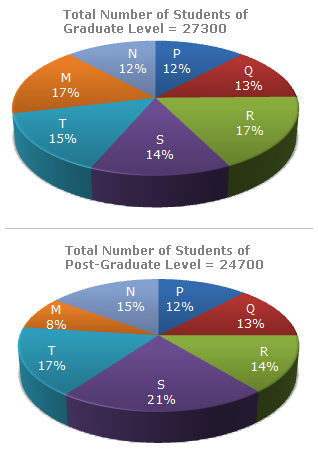# Data Interpretation - Pie Charts

### Exercise :: Pie Charts - Pie Chart 6

The following pie-charts show the distribution of students of graduate and post-graduate levels in seven different institutes in a town.1.

What is the total number of graduate and post-graduate level students is institute R?

 A. 8320 B. 7916 C. 9116 D. 8099

Explanation:

 Required number = (17% of 27300) + (14% of 24700) = 4641 + 3458 = 8099.

2.

What is the ratio between the number of students studying at post-graduate and graduate levels respectively from institute S?

 A. 14 : 19 B. 19 : 21 C. 17 : 21 D. 19 : 14

Explanation:

 Required ratio = (21% of 24700) = (21 x 24700) = 19 . (14% of 27300) 14 x 27300 14

3.

How many students of institutes of M and S are studying at graduate level?

 A. 7516 B. 8463 C. 9127 D. 9404

Explanation:

Students of institute M at graduate level= 17% of 27300 = 4641.

Students of institute S at graduate level = 14% of 27300 = 3822.Total number of students at graduate in institutes M and S = (4641 + 3822) = 8463.

4.

What is the ratio between the number of students studying at post-graduate level from institutes S and the number of students studying at graduate level from institute Q?

 A. 13 : 19 B. 21 : 13 C. 13 : 8 D. 19 : 13

Explanation:

 Required ratio = (21% of 24700) = (21 x 24700) = 19 . (13% of 27300) 13 x 27300 13

5.

Total number of students studying at post-graduate level from institutes N and P is

 A. 5601 B. 5944 C. 6669 D. 8372